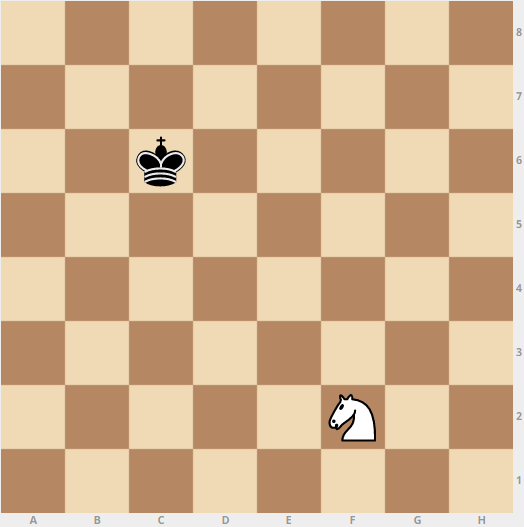# King And KnightDimitri places a Black King and a White Knight on 2 distinct squares in an empty chessboard. If the probability that Dimitri places the King and the Knight on the chessboard such that the King is not in check (that is, the Knight is not attacking the Black King), can be expressed as $\dfrac{m}{n}$, where $m$ and $n$ are coprime positive integers, find $m+n$.

As an explicit example, if the Knight is on $\text{f2}$, the $\text{h3}$, $\text{d3}$, $\text{d1}$, $\text{h1}$, $\text{g4}$ and $\text{e4}$ squares are under attack.

×